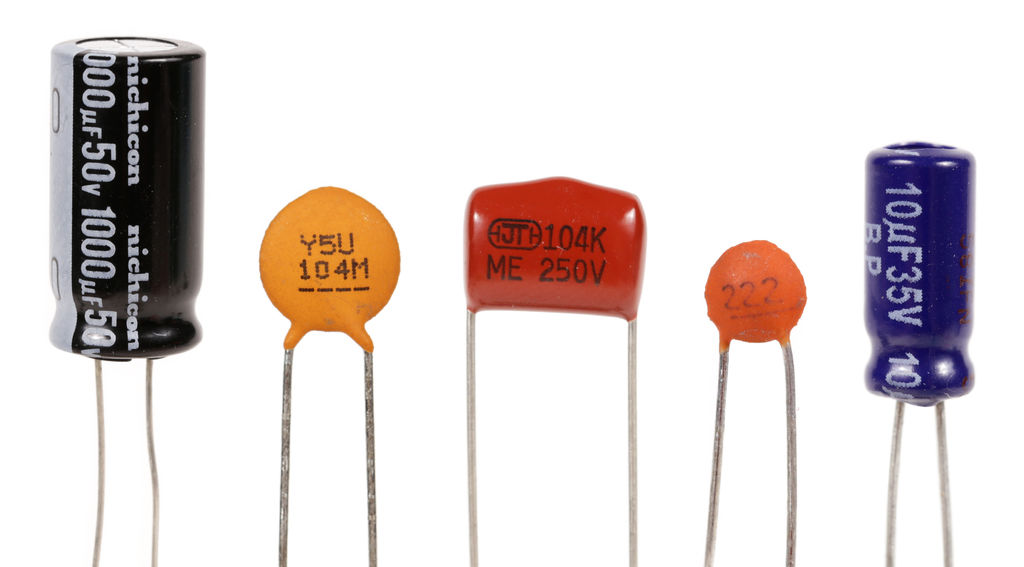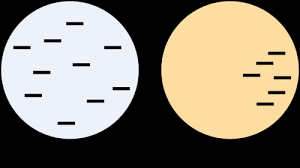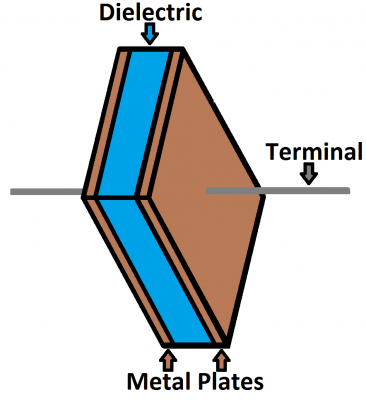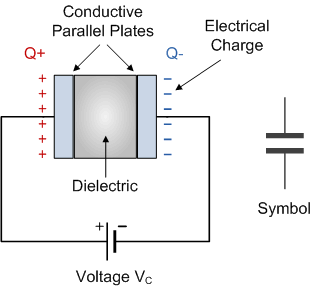# Capacitance Study Guide

INTRODUCTION

Have you ever looked at a Printed Circuit Board (PCB) and wondered what those tiny battery-like things are across the board? Well, those things are called capacitors, and similar to batteries, they can store some energy in the form of a charge that is essential for the functioning of a circuit. Unlike batteries which generate energy through a chemical reaction, these little energy devices work on a property called capacitance.Source

### WHAT IS A CAPACITOR?

We know that conductors are materials that allow the free flow of electrons through them and insulators are materials that do the opposite. However, when you place excess electrons on both materials, the distribution of electrons varies, as in the diagram below. On a conductor, the electrons repel each other and distribute themselves evenly over the surface; on an insulator, the electrons clump together in the same place since they cannot move about freely.Source

The capacitor is a charge storing device built like a sandwich of an insulator placed between two conducting plates. The insulator is called a dielectric, and its function is to boost the charging storing capacity of the capacitor.Source

The dielectric in a capacitor unit is made out of different insulating materials such as ceramic, paper, rubber, plastic, and other materials that impede the flow of electricity.

### WHAT IS CAPACITANCE?

When you apply an electric current, which is the flow of electric charge across the terminals of a capacitor, the charges get stuck on the capacitor plates. This is because the insulating dielectric won’t allow them to pass. As a result, negative charges start building upon one plate and push the like charges away on the other plate giving it a positive charge.Source

The opposite charges attract each other and stay stuck in the same place as the dielectric won’t allow them to come together. These stationary charges create an electric field and voltage like a battery. As the charging continues, the capacitor reaches a point where it can’t accept any more charge flowing into it as the existing charge starts repelling the incoming charge. This limit is where the concept of capacitance comes into play.

In simple terms capacitance is the maximum charge the capacitor can store. More technically, it is the ratio of maximum charge Q that the capacitor can hold to the applied voltage V across its plates.
Mathematically the capacitance formula is given by:

C = QV or Q = CV

Where,C is the capacitance measured in Fared (F)Q is the charge in Coulombs V is the voltage in volts

A capacitor is said to have a capacitance of one Fared when it can store one Coulomb of charge on its plates by a voltage of one Volt.

Microfarad (μF) 1μF = 1/1,000,000 = 0.000001 = 10-6 F

Nanofarad (nF) 1nF = 1/1,000,000,000 = 0.000000001 10-9 = F

Picofarad (pF) 1pF = 1/1,000,000,000,000 = 0.000000000001 10-12 = F

## CONCLUSION

• Capacitors are charge storing devices that are used in circuits.
• Capacitors can be charged by applying an electric current across its terminals.
• Positive and negative charges build up along the two plates of a capacitors.
• The capacitance definition physics is the ratio of maximum charge Q that the capacitor can hold to the applied voltage V across its plates.

### FAQs:

1. What is the difference between capacitor and capacitance?

A capacitor is a device which stores energy in the form of electric charge. Capacitance is the ability of a capacitor to store charges.

2. What is capacitor and its unit?

A capacitor uses two conducting plates with a dielectric in between to store charges. The unit of measuring a capacitor’s capacitance is Farad.

We hope you enjoyed studying this lesson and learned something cool about Capacitance! Join our Discord community to get any questions you may have answered and to engage with other students just like you! We promise, it makes studying much more fun! 😎

## SOURCES:

1. Capacitance. https://www.ck12.org/section/capacitance-%3a%3aof%3a%3a-electric-potential-worksheets-%3a%3aof%3a%3a-ck-12-physics-intermediate-workbook/. Accessed 22 Feb 2022.
2. Capacitors and Capacitance. https://phys.libretexts.org/Bookshelves/University_Physics/Book%3A_University_Physics_(OpenStax)/Book%3A_University_Physics_II_-_Thermodynamics_Electricity_and_Magnetism_(OpenStax)/08%3A_Capacitance/8.02%3A_Capacitors_and_Capacitance. Accessed 22 Feb 2022.
3. Capacitors. https://physics.info/capacitors/. Accessed 22 Feb 2022.
4. Capacitor And Capacitance. https://byjus.com/physics/capacitor-and-capacitance/#:~:text=The%20ability%20of%20the%20capacitor,with%20a%20gap%20between%20them. Accessed 22 Feb 2022.
]]>